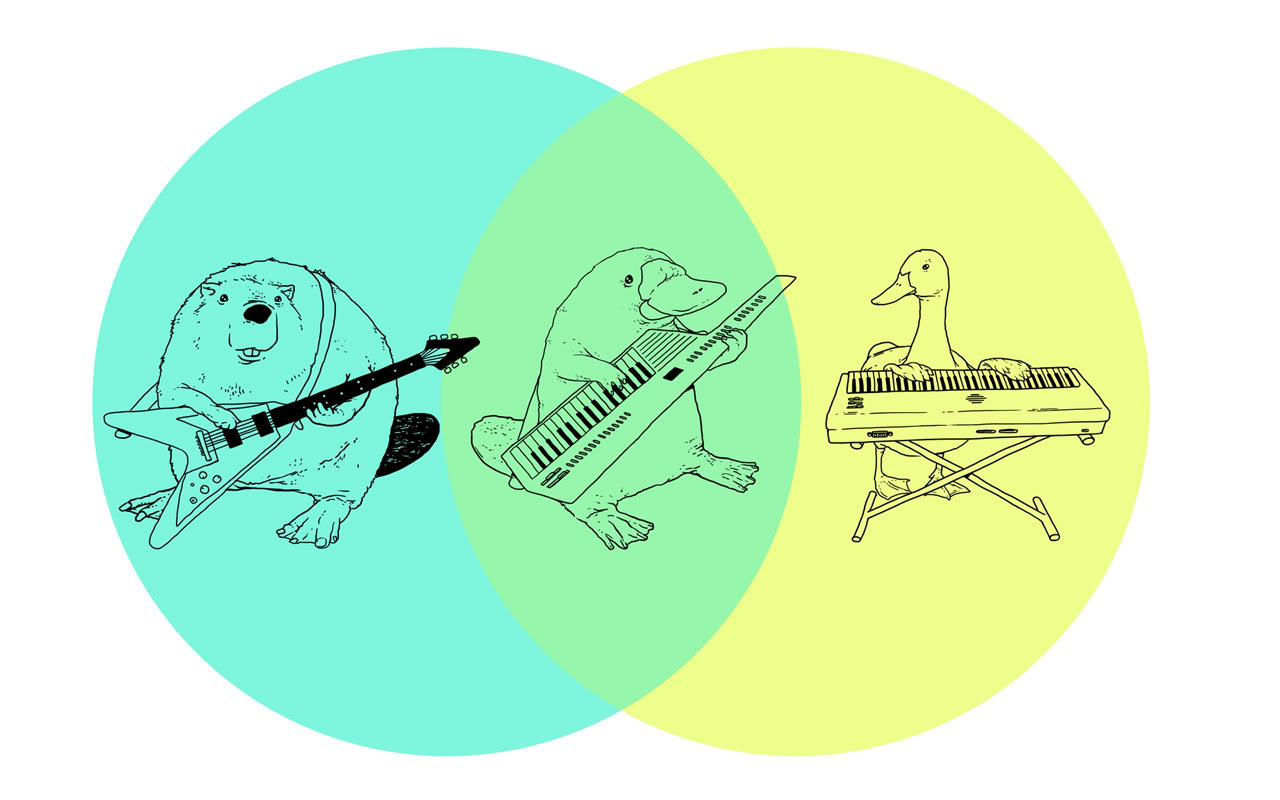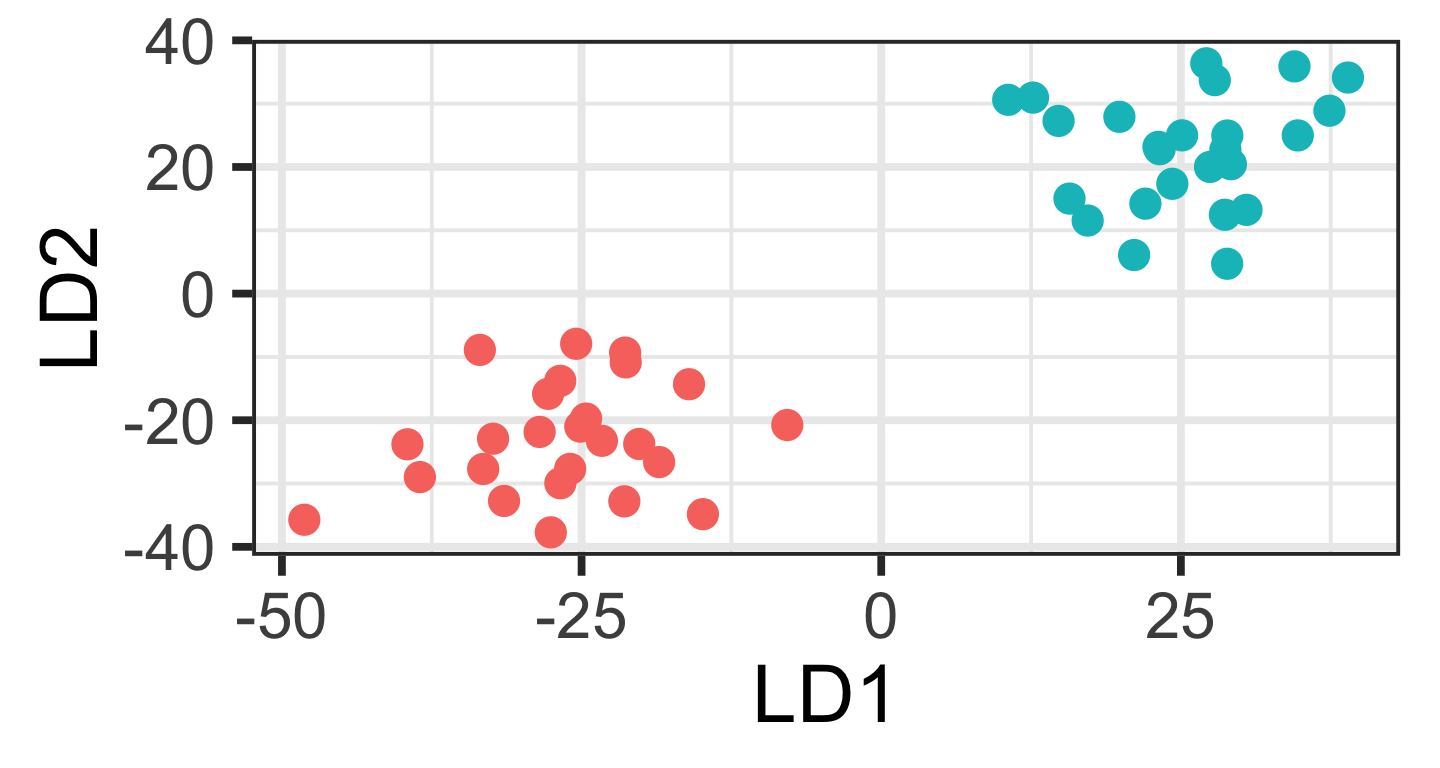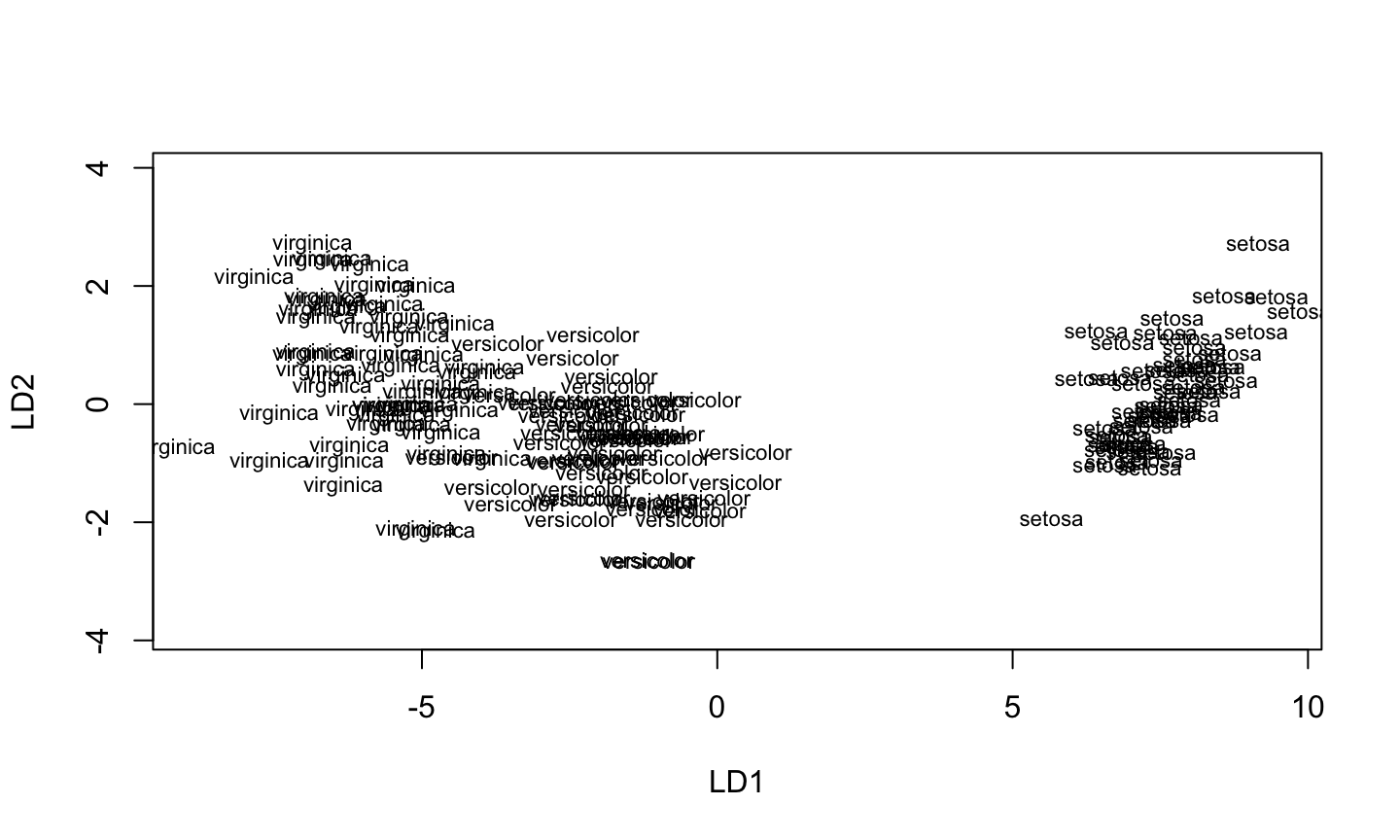## Multivariate Stats Continued## Discriminant Function Analysis

DFA finds a linear combination of the \(p\) variables that maximizes the distance between groups

\[Z = a_1X_1 + a_2X_2 + ... + a_pX_p\]

DFA tries to maximise the F ratio of between group to within group variation (\(M_B/M_W\))

This is an eigenvalue problem

## Discriminant Function Analysis

Assuming you have more measurements than groups, there will be \(m - 1\) canonical discriminant functions that maximize the ratio \(M_B/M_W\).

These are indicated by \(Z_1\), \(Z_2\), \(...\), \(Z_{m-1}\).

\(Z_1\) captures as much distance between groups as possible.

\(Z_2\) captures as much variation as possible, subject to the condition that the variation captured is uncorrelated (orthogonal) to \(Z_1\), and so on with the remaining canonical discriminant functions.

## Discriminant Function Analysis

First two discriminant functions often captures majority of group differences.

If so, we can use reduced set of variables to visualize \(p\) dimensional dataset in 2 dimensions.## DFA in R

```library(MASS)
DFA <- lda(Species ~ ., data=iris)
DFA```

Can anyone guess what the . does in this formula?

Results of the DFA are shown on the next page.

## DFA in R

```## Call:
## lda(Species ~ ., data = iris)
##
## Prior probabilities of groups:
##     setosa versicolor  virginica
##  0.3333333  0.3333333  0.3333333
##
## Group means:
##            Sepal.Length Sepal.Width Petal.Length Petal.Width
## setosa            5.006       3.428        1.462       0.246
## versicolor        5.936       2.770        4.260       1.326
## virginica         6.588       2.974        5.552       2.026
##
## Coefficients of linear discriminants:
##                     LD1         LD2
## Sepal.Length  0.8293776  0.02410215
## Sepal.Width   1.5344731  2.16452123
## Petal.Length -2.2012117 -0.93192121
## Petal.Width  -2.8104603  2.83918785
##
## Proportion of trace:
##    LD1    LD2
## 0.9912 0.0088```

## DFA in R

`plot(DFA)`## DFA in R

The LD scores for each point are not stored in the DFA object. To get these, we need to use the `predict()` function.

```predictions <- predict(DFA)
```##        LD1        LD2
## 1 8.061800  0.3004206
## 2 7.128688 -0.7866604
## 3 7.489828 -0.2653845
## 4 6.813201 -0.6706311
## 5 8.132309  0.5144625
## 6 7.701947  1.4617210```
`head(predictions\$class)`
```##  setosa setosa setosa setosa setosa setosa
## Levels: setosa versicolor virginica```
`head(predictions\$posterior)`
```##   setosa   versicolor    virginica
## 1      1 3.896358e-22 2.611168e-42
## 2      1 7.217970e-18 5.042143e-37
## 3      1 1.463849e-19 4.675932e-39
## 4      1 1.268536e-16 3.566610e-35
## 5      1 1.637387e-22 1.082605e-42
## 6      1 3.883282e-21 4.566540e-40```

## DFA in R

The `predict()` function is also used to classify additional cases that did not go into the original discriminant function analysis

```newCases <- data.frame(
Sepal.Length = c(4.5, 6.7),
Sepal.Width = c(3.5, 2.5),
Petal.Length = c(1.5, 4),
Petal.Width = c(.75, 2)
)```

## DFA in R

`predict(DFA, newdata = newCases)`
```## \$class
##  setosa     versicolor
## Levels: setosa versicolor virginica
##
## \$posterior
##         setosa   versicolor    virginica
## 1 1.000000e+00 1.852455e-13 5.856421e-29
## 2 3.781122e-24 9.058903e-01 9.410972e-02
##
## \$x
##         LD1       LD2
## 1  5.798299 1.7543205
## 2 -2.927648 0.8620058```

## Correspondence Analysis

• A method for visualizing a 2-way contingency table
• The goal is to have rows (often taxa) and colums (often sites) appear in same ordination plot
• Often called reciprocal averaging
• Site scores are weighted averages of species values, and species scores are a weighted average of site values
• useful for count data and presence/absence

## Correspondence Analysis

```bovids <- read.table("http://hompal-stats.wabarr.com/datasets/bovid_occurrences.txt", header=TRUE, sep="\t")
library(tidyr)
row.names(bovids) <- bovids\$taxon
bovids<-bovids[,2:9]
```##              site1 site2 site3 site4 site5 site6 site7 site8
• `ca` package in R